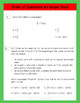# 5th Grade Place Value, Computations, Order of Operations and Word Problems GamesSubject
Resource Type
File Type

Zip

(22 MB|21 pages)
Product Rating
4.0
(1 Rating)
Standards
• Product Description
• StandardsNEW

Download this product and get access to over 25 questions covering place value, estimation/rounding, order of operations, multiplication, and division computations, and word problems! This resource has it ALL! Use the games included in your small group teacher table, in a workshop, at home with your child, or as a whole group activity. Answer key and game pieces included. Let's make learning TEK/Common Core aligned, challenging, AND fun! This resource does all THREE!

Interpret the product (𝘢/𝘣) × 𝘲 as a parts of a partition of 𝘲 into 𝘣 equal parts; equivalently, as the result of a sequence of operations 𝘢 × 𝘲 ÷ 𝘣. For example, use a visual fraction model to show (2/3) × 4 = 8/3, and create a story context for this equation. Do the same with (2/3) × (4/5) = 8/15. (In general, (𝘢/𝘣) × (𝘤/𝘥) = 𝘢𝘤/𝘣𝘥.)
Write simple expressions that record calculations with numbers, and interpret numerical expressions without evaluating them. For example, express the calculation “add 8 and 7, then multiply by 2” as 2 × (8 + 7). Recognize that 3 × (18932 + 921) is three times as large as 18932 + 921, without having to calculate the indicated sum or product.
Use parentheses, brackets, or braces in numerical expressions, and evaluate expressions with these symbols.
Fluently multiply multi-digit whole numbers using the standard algorithm.
Use place value understanding to round decimals to any place.
Total Pages
21 pages
Included
Teaching Duration
30 minutes
Report this Resource to TpT
Reported resources will be reviewed by our team. Report this resource to let us know if this resource violates TpT’s content guidelines.